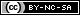# Migrate

Migrate: MIGRATION RATE AND POPULATION SIZE ESTIMATION using Markov Chain Monte Carlo simulation

This application can get occasionally caught in an infinite loop during startup and fill your alloted space on a filesystem. To avoid this problem, please ask the program to write a parmfile. Then use only the parmfile to run again, instead of using the input. This requires killing the job and then starting again using the parmfile.

The infinite loop problem looks like this:

=============================================
MIGRATION RATE AND POPULATION SIZE ESTIMATION
using Markov Chain Monte Carlo simulation
=============================================
PDF output enabled [Letter-size] Version 3.0.3
Program started at Fri Sep 11 12:19:45 2009

Settings for this run:
D Data type currently set to: DNA sequence model
I Input/Output formats
P Parameters [start, migration model] S Search strategy
W Write a parmfile
Q Quit the program

Are the settings correct?
(Type Y or the letter for the menu to change)
===>
PARAMETERS
---------------------------
Start parameters:
1 Use a simple estimate of theta as start?
Estimate with FST (Fw/Fb) measure
2 Use a simple estimate of migration rate as start?
Estimate with FST (Fw/Fb) measure

Gene flow parameter and Mutation rate variation among loci:
3 Use M for the gene flow parameter YES [M=m/mu] 4 Mutation rate is Constant

FST-Calculation (for START value):
5 Method: Variable M, Theta is the same for all populations
6 Print FST table: NO

Migration model:
7 Model is set to Full migration matrix model
8 Geographic distance matrix: NO

Are the settings correct?
(Type Y to go back to the main menu or the letter for an entry to change)
===> PARAMETERS
---------------------------
Start parameters:
1 Use a simple estimate of theta as start?
Estimate with FST (Fw/Fb) measure
2 Use a simple estimate of migration rate as start?
Estimate with FST (Fw/Fb) measure

Gene flow parameter and Mutation rate variation among loci:
3 Use M for the gene flow parameter YES [M=m/mu] 4 Mutation rate is Constant

FST-Calculation (for START value):
5 Method: Variable M, Theta is the same for all populations
6 Print FST table: NO

Migration model:
7 Model is set to Full migration matrix model
8 Geographic distance matrix: NO

Are the settings correct?
(Type Y to go back to the main menu or the letter for an entry to change)
===> Which method? (F)st or (O)wn or (N)ormally or ((U)niform distributed
random value
===> PARAMETERS
---------------------------
Start parameters:
1 Use a simple estimate of theta as start?
Estimate with FST (Fw/Fb) measure
2 Use a simple estimate of migration rate as start?
Estimate with FST (Fw/Fb) measure

Gene flow parameter and Mutation rate variation among loci:
3 Use M for the gene flow parameter YES [M=m/mu] 4 Mutation rate is Constant

FST-Calculation (for START value):
5 Method: Variable M, Theta is the same for all populations
6 Print FST table: NO

Migration model:
7 Model is set to Full migration matrix model
8 Geographic distance matrix: NO

Are the settings correct?
(Type Y to go back to the main menu or the letter for an entry to change)
===> Which FST calculation method?
(T)heta can be different for each population
and migration rates are symmetric.
(Number of populations >= 2)
(M)igration rate can be asymmetric
and Theta is the same for both populations
(Number of populations = 2)
===> PARAMETERS
---------------------------
Start parameters:
1 Use a simple estimate of theta as start?
Estimate with FST (Fw/Fb) measure
2 Use a simple estimate of migration rate as start?
Estimate with FST (Fw/Fb) measure

Gene flow parameter and Mutation rate variation among loci:
3 Use M for the gene flow parameter YES [M=m/mu] 4 Mutation rate is Constant

FST-Calculation (for START value):
5 Method: Variable Theta, M symmetric
6 Print FST table: NO

Migration model:
7 Model is set to Full migration matrix model
8 Geographic distance matrix: NO

Are the settings correct?
(Type Y to go back to the main menu or the letter for an entry to change)
===> Specify the migration model as an {n x n} matrix
Theta values are on the diagonal, migration rates are
off-diagonal, spaces (" "), "{", "}", or newlines
are allowed, but not necessary.

Syntax:
* = independent parameter
0 = (zero) not estimated] c = (constant) not estimated, taken from start-parameter] s = symmetric migration rates (M=m/mu)
S = symmetric migration rates (xNm)
m = average of each label group [not k, or s] How many populations?
===> How many populations?
===>
You must give 225 values
===> Enter the next value or list of values
Enter the next value or list of values
Enter the next value or list of values
Enter the next value or list of values
Enter the next value or list of values
Enter the next value or list of values
Enter the next value or list of values
Enter the next value or list of values
Enter the next value or list of values
Enter the next value or list of values
Enter the next value or list of values
Enter the next value or list of values
Enter the next value or list of values
Enter the next value or list of values
Enter the next value or list of values
Enter the next value or list of values
Enter the next value or list of values

this repeats indefinitely...This work is licensed under a Creative Commons Attribution-NonCommercial-ShareAlike 4.0 International License. Permissions beyond the scope of this license may be available at Attribution.